• 给定2维样本500个，存放在文件“500样本.txt”中，其中前300个是属于第一类的样本，接着200个是属于第...假设类样本均来自正态总体，试分别估计参数，求出决策函数和决策规则并对如下五个未知类别的样本进行分类
• 第三章最大似然估计和贝叶斯参数估计 3.1引言 3.2最大似然估计 3.3贝叶斯估计 3.4贝叶斯参数估计 3.5贝轩斯参数估计一般理论 3.6充分统计论 3.7维数问题 3.8成分分析和判别函数 3.9期望最大化算法 3.10隐...matlab
• 第三章最大似然估计和贝叶斯参数估计 3.1引言 3.2最大似然估计 3.3贝叶斯估计 3.4贝叶斯参数估计 3.5贝轩斯参数估计一般理论 3.6充分统计论 3.7维数问题 3.8成分分析和判别函数 3.9期望最大化算法 3.10隐...matlab
• 算法简介KNN(K近邻算法)是一种不需要学习任何参数同时也非常简单的机器学习算法，既可以用来解决分类问题也可以用来解决回归问题。直观解释这个算法就是'近朱者赤，近墨者黑'，当输入一个新样本时，根据与其相近的...


算法简介KNN(K近邻算法)是一种不需要学习任何参数同时也非常简单的机器学习算法，既可以用来解决分类问题也可以用来解决回归问题。直观解释这个算法就是'近朱者赤，近墨者黑'，当输入一个新样本时，根据与其相近的样本值来估计新输入的样本。如下图所示新输入的样本会被分类为W1。影响算法的几个因子在了解算法大体的思路后，其实还有几个问题需要继续深究:1、如何表达两个样本的距离(相似度)？ 2、KNN中的K值应该如何选取？ 3、确定了相邻的点后，如何得出最终的结果？距离的计算:首先我们把每个样本的特征映射到特征空间中,样本距离的衡量其实就是空间中两点之间的距离，一般使用的是欧式距离使用哪种方式来计算距离还是需要看具体的场景以及要解决的问题(domain-knowledge)，比如输入经纬度的信息预测房价，计算距离的话可以直接使用经纬度的距离计算公式。预测黑色点的房价K值的选取:K值的选择会对最后的结果产生非常大的影响，假设我们选取的k值很小那么样本很容易被周围的异常点给影响，这样的模型容易过拟合。下图为是k取1时的效果图，很明显边界非常不平滑并且一些异常点严重影响到了分类的结果。K取值为1的分类效果 那如果选择较大的K值，就相当于用较大范围的训练实例进行预测，可以减少异常数据的影响。假如K取值为整个样本的大小那么最后的结果相当于对样本整体做了个平均，这时模型会过于简单。总结一下：K取值越小，模型越容易过拟合。取值越大，模型越简单，发现不了数据的差异。确定相邻点后的的决策过程:一般情况下直接对K个值取平均或者多数表决来决定最后的预测结果，也可以对每种类型或者不同距离的远近施加不同的权重，具体需要根据业务场景来决定。算法实现:下面是一个非常简单的版本实现，每次都需要遍历完一遍样本集，最后取平均得出预测结果。import numpy as npfrom sklearn.neighbors import KNeighborsClassifierfrom sklearn.metrics.pairwise import pairwise_distances‘’‘# samples为需要分类的样本# topk为Number of neighbors’‘’def predict_by_k_nearest(samples, features, labels, topk):    distance = [pairwise_distances(i, samples) for i in features]    index = np.argsort(distance)[:topk]    return 1 if (y[index].mean() > 0.5) else 0完成分类过程，以sklearn的KNeighborsClassifier做了一下验证，发现预测结果都是1。sklearn的KNeighborsClassifier有不同的参数可以设置，但都围绕我们之前讨论的3个问题。X = np.asarray([, , , , ])y = np.asarray([0, 0, 1, 1, 1])neigh = KNeighborsClassifier(n_neighbors=3,weights='uniform')neigh.fit(X, y)print(neigh.predict([]))print (predict_by_k_nearest(, X, y, 3))### 补充：KNN每次在计算距离的时候都需要遍历完全部的样本，非常耗时。我们可以使用KD树来优化查询的过程，核心思想就是把相近的样本索引在同一个区间上，这样每次查询的时候只要看相近的区间都可以。这部分我没有实现，有兴趣的同学可以在网上查询相关的资料。∞∞∞∞∞IT派 - {技术青年圈}持续关注互联网、区块链、人工智能领域公众号回复“机器学习”，邀你加入{ IT派AI机器学习群 }


展开全文• Logistic Regression解决二分类问题 一、实验目的 理解逻辑回归模型，掌握逻辑回归模型的参数估计算法。 二、实验要求实验环境 要求： 实现种损失函数的参数估计（1，无惩罚项；2.加入对参数的惩罚），可以采用...
Logistic Regression解决二分类问题
一、实验目的
理解逻辑回归模型，掌握逻辑回归模型的参数估计算法。
二、实验要求及实验环境
要求：
实现两种损失函数的参数估计（1，无惩罚项；2.加入对参数的惩罚），可以采用梯度下降、共轭梯度或者牛顿法等。
实验环境：
windows10、python3.7.4、pycharm2020.2
三、数学原理
3.1	实验目的及假设
实验目的：
从$Training Set：,...$中学习到一个分类器
$f:X\to Y$
以便于预测一个新的样本$X^{new}$所属的类别$label$
实验假设：

$X$的每一维属性$X_i$都是实数，故$X$可视为形如$$的$n$维$vector$

$Y$是$boolean$值，取值为1或0

$X_i$关于$Y$条件独立

$P(X_i| Y=y_k)\sim N(\mu_{ik},\sigma_{i})$

$P(Y)\sim B(\pi)$

3.2	转化$P(Y|X)$
3.2.1	利用实验假设
按照前面的实验假设，结合概率论的知识，我们可以得到：
\begin{aligned} P(Y=1|X)&=\frac{P(Y=1)P(X|Y=1)}{P(Y=1)P(X|Y=1)+P(Y=0)P(X|Y=0)}\\ &=\frac{1}{1+\frac{P(Y=0)P(X|Y=0)}{P(Y=1)P(X|Y=1)}}\\ &=\frac{1}{1+exp(ln\frac{P(Y=0)P(X|Y=0)}{P(Y=1)P(X|Y=1)})}\\ &=\frac{1}{1+exp(ln\frac{1-\pi}{\pi}+\sum_iln\frac{P(X_i|Y=0)}{P(X_i|Y=1)})} \end{aligned}
由于$P(X_i|Y=y_k)=\frac{1}{\sigma_{i}\sqrt{2\pi}}exp(\frac{-(X_i-\mu_{ik})^2}{2\sigma_{i}^2})$
代回原来的式子，可得
$P(Y=1|X)=\frac{1}{1+exp(w_0+\sum_{i=1}^nw_iX_i)} \\ w_0=\sum_i\frac{\mu_{i1}^2-\mu_{i0}^2}{2\sigma_i^2}+ln\frac{1-\pi}{\pi}; w_i=\frac{\mu_{i0}-\mu_{i1}}{\sigma_i^2}$
因此
\begin{aligned} P(Y=0|X)&=\frac{exp(w_0+\sum_{i=1}^nw_iX_i)}{1+exp(w_0+\sum_{i=1}^nw_iX_i)}\\ ln \frac{P(Y=0|X)}{P(Y=1|X)}&=ln(exp(w_0+\sum_{i=1}^nw_iX_i))=w_0+\sum_{i=1}^nw_iX_i \end{aligned}
3.2.2	引入odds
这里使用了一个分类的思想：利用$odds$

一个事件的几率$odds$是指事件发生的概率与事件不发生的概率的比值，如果事件发生的概率是$p$，那么该事件的几率$odds=\frac{p}{1-p}$，该事件的对数概率（logit函数）就是

$logit(p)=ln \frac{p}{1-p}$

依据$logit(p)$>0还是<0，来判定事件发生还是不发生，这便是$odds$概念的作用

将其应用到我们的$Logistic Regression$问题中来便是
$logit(Y=0|X)=w_0+\sum_{i=1}^nw_iX_i$
若$logit(Y=0|X)>0$则将$X$分到$Y=0$类，若$logit(Y=0|X)<0$则将$X$分到$Y=1$类。
故我们的类别分界线就是
$w_0+\sum_{i=1}^nw_iX_i=0$
将其向量化
$w^TX=0\\ w=[w_0,w_1...w_n],X=[1,X_1,X_2...X_n]$
注意这里的$w$和$X$都是$n+1$维向量，拓展了一个维度。
现在，还可以量化求出$X$属于$Y=1$类和$Y=0$类的概率
$P(Y=1|X)=\frac{1}{1+exp(w^TX)}=sigmoid(-w^TX)$
$P(Y=0|X)=\frac{exp(w^TX)}{1+exp(w^TX)}=\frac{1}{1+exp(-w^TX)}=sigmoid(w^TX)$
3.2.3	引入sigmoid函数
注意，这里我们引入了一个$sigmoid$函数，图像如下：所谓sigmoid函数，是一个在生物学中常见的S型函数，也称为S型生长曲线；也常常运用于信息科学当中，由于其单增以及反函数单增等性质，Sigmoid函数常被用作神经网络的激活函数，将变量映射到0,1之间。
它是一个从实数域到(0,1)区间的映射，可以用来做二分类。在特征相差比较复杂或是相差不是特别大时效果比较好。Sigmoid作为激活函数有以下优点：
平滑、易于求导。

我们在这里利用$sigmoid(w^TX)$来表示$P(Y=0|X)$，既满足$w^TX$是在实数域上，又满足$sigmoid(w^TX)$是在$(0,1)$区间上，且该函数光滑可导，十分契合我们的需求。
3.2.4	总结
我们得到的$X$属于2种类别的分界线是
$w^TX=0 \tag{1}$
当$w^TX>0$时认为$X$属于$Y=0$类；若$w^TX<0$则认为$X$属于$Y=1$类。
而把$X$归类为2种类别的概率分别是
$P(Y=1|X)=sigmoid(-w^TX) \tag{2}$
$P(Y=0|X)=sigmoid(w^TX) \tag{3}$
3.3	找到loss函数
前面我们已经得到了分类的界限$w^TX=0$，那么我们该如何确定这里的参数$w$呢？
有两种方法：最大似然估计MLE和贝叶斯估计MAP，两者在$loss$函数里面就分别代表了无正则项的loss函数和有正则项的loss函数。
3.3.1	用MCLE求解$w$

MLE的核心思想就是：将参数$w$看作唯一真值，我们的任务就是找到这个$w$，使得在这组参数下，我们的数据的似然度（概率）最大。

也就是说我们需要求$P(|w)$，但这是很困难的事情，于是我们可以将MLE转换为MCLE，只需要计算 $P(Y|X，w)$
于是我们的似然函数就是
\begin{aligned} L(w)&=ln\prod_l P(Y^l|X^l,w)\\ &=\sum_l(Y^lw^TX^l-ln(1+exp(w^TX^l))) \end{aligned}
我们的损失函数$loss(w)$一般取$-L(w)$，即
$loss(w)=\sum_l(-Y^lw^TX^l+ln(1+exp(w^TX^l))) \tag{4}$
注意：这里的$X^l和Y^l$均表示第$l$个样本
3.3.2	用MAP求解$w$

MAP的核心思想是：$w$是一个随机变量，符合一定的概率分布。
所以我们的任务就是给$w$添加一个先验$P(w)$，然后使得$P(w)P(Y|X,w)$最大。

我们假设$w_i\sim N(0,\sigma)$，则似然函数为
$L(w)=\sum_l(Y^lw^TX^l-ln(1+exp(w^TX^l)))-\frac{w^Tw}{2\sigma^2}+ln(\frac{1}{\sqrt{2\pi}\sigma})$
简化为
$L(w)=\sum_l(Y^lw^TX^l-ln(1+exp(w^TX^l)))-\frac{\lambda}{2}w^Tw$
则MAP情况下的$loss$函数为
$loss(w)=\sum_l(-Y^lw^TX^l+ln(1+exp(w^TX^l)))+\frac{\lambda}{2}w^Tw \tag{5}$
相当于在MLE的基础上加了正则项
3.4	求出loss函数的优化解——牛顿法
前面我们已经找出了MLE和MAP情况下的$loss$函数，我们所需的$w$为
$w=\operatorname{argmin}_{w}loss(w) \tag{6}$
我们使用牛顿法来求解

牛顿法的思路是使用二阶泰勒展开去估计曲线，然后用二阶泰勒展开的函数的极值点去估计曲线的极值点，重复迭代直到找到极值点对于无约束最优化问题
$min_{x}f(x)$
其中$x^*$为函数极小值点
设$f(x)$有二阶连续偏导数，若第$k$次迭代值为$x^k$，则可以将$f(x)$在$x^k$附近进行二阶泰勒展开
$f(x)=f(x^k)+g_k^T(x-x^k)+\frac{1}{2}(x-x^k)^TH(x^k)(x-x^k) \tag{7}$
其中，$g_k=\bigtriangledown f(x)$是梯度向量，$H(x)$是海森矩阵
$H(x)=[\frac{\partial^2 f}{\partial x_i\partial x_j}]_{n\times n}$
$f(x)$在极值点处$g_k=0$；特别的，当$H(x)$为正定矩阵时，$f(x)$的极值是极小值。
为了得到$g_k=0$的点，对7式求导
$\frac{df(x)}{dx}=g_k+H_k(x-x^k)$
则极值点处$\frac{df(x)}{dx}=0$
$x^{k+1}=x^k-H_k^{-1}g_k$
这便是我们的迭代公式。
将其应用到我们的$loss$函数就是
$w^{k+1}=w^k-(\frac{\partial^2 loss(w)}{\partial w\partial w^T})^{-1}\frac{\partial loss(w)}{\partial w} \tag{8}$
其中
$\frac{\partial loss(w)}{\partial w}=-\sum_lx^l(Y^l-sigmoid(w^TX))+\lambda w \tag{9}$
$\frac{\partial^2 loss(w)}{\partial w\partial w^T}=\sum_l(XX^Tsigmoid(w^TX)sigmoid(-w^TX))+\lambda I \tag{10}$
注意：上面的式子对于MLE的$loss$函数而言$\lambda$=0，$I$表示单位阵
四、实验具体流程
4.1	生成数据
设置正例($Y=1$)的比例为40%，训练集、验证集、测试集的比例为6：2：2
利用多维高斯分布函数来生成数据，为便于画图展示，主要使用二维数据。
4.1.1	满足朴素贝叶斯
若满足朴素贝叶斯，则认为$X$的各维度数据关于$Y$条件独立，则协方差矩阵为
$C=\begin{bmatrix} cov_{11} &cov_{12} \\ cov_{21} &cov_{22} \end{bmatrix}$
其中$cov_{12}=cov_{21}=0$，$cov_{11}$就是$X_1$的方差，$cov_{22}$就是$X_2$的方差，故
$C=\begin{bmatrix} \sigma_1^2 &0 \\ 0 &\sigma_2^2 \end{bmatrix}$
自己设定$\sigma_1^2=0.3,\sigma_2^2=0.4$
展示核心代码：
    def Data(N, naive=True, posRate=0.4):
posNumber = np.ceil(N * posRate).astype(np.int32)
sigma = [0.3, 0.4]  # cov11与cov22
cov12 = 0.2
pos_mean = [1, 1.2]  # 正例的两维度均值
neg_mean = [-1, -1.2]  # 反例的两维度均值
x = np.zeros((N, 2))  # x数组
y = np.zeros(N).astype(np.int32)  # label数组
if naive:  # 满足朴素贝叶斯假设
x[:posNumber, :] = np.random.multivariate_normal(pos_mean, [[sigma, 0], [0, sigma]],
size=posNumber)
x[posNumber:, :] = np.random.multivariate_normal(neg_mean, [[sigma, 0], [0, sigma]],
size=N - posNumber)

y[:posNumber] = 1
y[posNumber:] = 0

实验效果如下4.1.2	不满足朴素贝叶斯
不满足朴素贝叶斯时，则$cov_{12} \ne 0$，自己设定$cov_{12}=0.2$
核心代码如下：
    else:  # 不满足朴素贝叶斯假设
x[:posNumber, :] = np.random.multivariate_normal(pos_mean, [[sigma, cov12], [cov12, sigma]],
size=posNumber)
x[posNumber:, :] = np.random.multivariate_normal(neg_mean, [[sigma, cov12], [cov12, sigma]],
size=N - posNumber)
y[:posNumber] = 1
y[posNumber:] = 0

效果如下可以明显发现：不满足朴素贝叶斯假设时，数据点呈现“长条”状，这表明$X$的2个维度之间有线性相关关系，与我们的预期想契合。
4.2	有无正则项的对比
在无正则项的时候，依据lab1的结论，当训练集数据点数据很少时，会有过拟合现象。然后克服过拟合的方法有2种：

增加训练集的样本数量
增加正则项

下面我们按照这个思路来进行有无正则项的对比实验
先展示一下Newton法求优化解的核心代码，至于算法的数学原理前面已经给出，这里不再赘述
 	def __derivative(self, w):
"""
求出导函数
:param w: 当前的w
:return: 返回当前的导数
"""
result = np.zeros(self.__n)
# 依次取出X和Y的所有行
for i in range(self.__m):
result += (self.x[i] * (self.y[i] -
(1.0 - self.__sigmoid(w @ self.x[i]))))
return -1 * result + self.hyper * w

def __second_derivative(self, w):
"""
求出hessian matrix的逆矩阵
:param w:
:return:
"""
ans = np.eye(self.__n) * self.hyper
# 依次取出X和Y的所有行
for i in range(self.__m):
temp = self.__sigmoid(w @ self.x[i])
ans += self.x[i] * np.transpose([self.x[i]]) * temp * (1 - temp)
# 最后求逆矩阵
return np.linalg.pinv(ans)

def solve(self):
w = self.w_0
while True:
gradient = self.__derivative(w)
# 满足精度要求即可退出迭代
if np.linalg.norm(gradient) < self.delta:
break
# 使用迭代公式进行下一次迭代
w = w - self.__second_derivative(w) @ gradient
return w

首先使用样本数量很少的训练集来训练，然后将训练得到的结果$w$应用到一个较大的测试集上测试它的泛化性能
4.2.1	无正则
我们仅仅使用$N=10$的小训练集：$Newton$法迭代情况如下，可见$Newton$法收敛还是比较快的：使用$N=2000$的测试集来测试它的泛化性能，正确率为89.75%：接下来使用更大的训练集$N=1000$：对应的收敛情况如下，可见在样本数较大时，迭代轮数并未受到太大影响。但是大训练集可以有效克服过拟合，此处准确率为99.45%4.2.2	有正则
使用一样的$N=10$的训练集，但是这次加上正则项，并先设定$\lambda=0.1$，正确率为98.65%：正确率大幅提高，这也与再一次证明了加入正则项可以克服过拟合的结论
收敛情况如下，可见增加正则项也不会明显改变迭代轮数：至于正则项的直观作用，可以参看下图即：正则项会改变求得的$w$，从而导致分界线的斜率和截距变化，具有更好的泛化性能。
至于正则项的超参数$\lambda$，我通过给它设定一个范围，然后在验证集上进行测试，选择泛化性能最好的超参数
$\lambda=exp(-3)$
并在后续实验中均使用此值4.3	是否满足朴素贝叶斯的对比
在数学原理部分已经叙述过，不满足朴素贝叶斯假设时协方差矩阵就不是对角阵，下面皆使用$N=1000,\lambda=exp(-3)$进行实验
满足朴素贝叶斯时:不满足朴素贝叶斯时，我们使用$cov_{12}=0.2$进行测试：分别进行10次实验，记录各自准确率：

1
2
3
4
5
平均

满足
0.996
0.994
0.995
0.994
0.992
0.994

不满足
0.972
0.976
0.974
0.973
0.968
0.973

可见，在其他条件相同时，”不满足朴素贝叶斯“的准确率略低于”满足朴素贝叶斯“
原因就在于：我们实验使用的是Logistic Regression，得到的分类器$w^TX=0$是个线性分类器，它只是给$X$的每个维度加个权重$w_i$，并没有考虑到各个维度之间的相关性，即默认满足了朴素贝叶斯假设。
4.4	使用UCI数据集进行测试
4.4.1	选用Skin数据集

Skin数据集：通过从各种年龄组（年轻人，中年人和老年人），种族组（白人，黑人和亚洲人）的面部图像中随机抽取B，G，R值以及从FERET数据库和PAL数据库获得的性别来收集皮肤数据集。
学习样本总量为245057; 其中50859是皮肤样本，194198是非皮肤样本。

此数据集中$X$有3个维度，$Y$有2种取值。正好适合我们进行二分类任务，同时由于$X$有3个维度，因此可以将其在3D图种显示出来。
从UCI上获取的数据集首先要读取出$X$和$Y$
def ReadUCI(path):
data_set = pd.read_csv(path)  # linux 相对路径
x = data_set.drop('label', axis=1)
y = data_set['label']
dataX, dataY = np.array(x, dtype=float), np.array(y)
N = len(dataY)
posNumber = 0
for i in range(N):
if dataY[i] == 1:
posNumber += 1

# 调节读取的数据量
useRate = 0.5
posUseNumber = int(math.ceil(posNumber * useRate))
negUseNumber = int(math.ceil((N-posNumber) * useRate))

x_Bool = np.zeros(N).astype(np.int32)  # x对应的bool数组，用于切片
x_Bool[:posUseNumber] = 1
x_Bool[posNumber:(posNumber + negUseNumber)] = 1
dataX, dataY = dataX[x_Bool == 1], dataY[x_Bool == 1]

# 划分训练集和测试集
Train_x, Train_y, Test_x, Test_y = SplitData(dataX, dataY)
return Train_x, Train_y, Test_x, Test_y

无正则项时：因为从正面看分界不是很清晰，所以我们找到分界的视角再看一下可见在不加正则项的时候，准确率也还不错，达到93.48%
加正则项时：同样展示分界面：可见：加入正则项会使得准确率略微上升，达到了95%
选用iris数据集

iris数据集中每个样本中$X$有4个维度，$Y$有3种取值。
于是我先将数据集进行处理，只留下$Y$有2种取值的那部分，以便于我们直接进行二分类。处理之后，数据集中共有100个样本点。在此数据集上进行测试：可见：准确率为100%
五、结论

对于在训练集样本数很少时，加入正则项可以有效解决过拟合问题。

类条件分布在满足朴素贝叶斯假设时的Logistic Regression分类表现，要比不满足假设时略好

Logistics Regression可以很好地解决简单的线性分类问题

使用牛顿法求优化解时，收敛速度较快，且样本点数目对它的收敛速度影响不大。

六、参考文献

周志华 著. 机器学习, 北京: 清华大学出版社, 2016.1
李航 著. 统计学习方法, 北京: 清华大学出版社, 2019.5


展开全文机器学习 python pycharm
• 第六章讲逻辑斯蒂回归模型和最大熵模型，这个模型都属于对数线性模型。...二项逻辑斯蒂回归模型是一种分类模型，由条件分布P(X|Y)表示，形式为参数化的逻辑斯蒂回归。这里，随机变量X取值为实数，随机变量Y取值为
第六章讲逻辑斯蒂回归模型和最大熵模型，这两个模型都属于对数线性模型。这两个模型学习一般采用极大似然估计，或正则化的 极大似然估计。逻辑斯蒂回归模型及最大熵模型学习可以转化为为无约束最优化问题。求解该最优化问题的算法有改进的迭代尺度法，梯度下降法，拟牛顿法。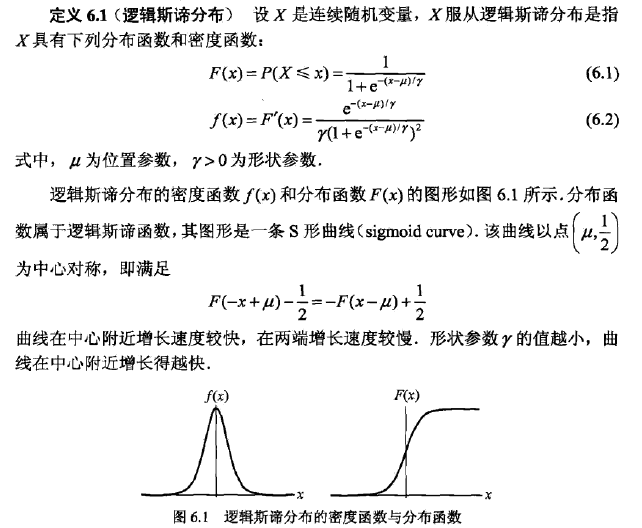二项逻辑斯蒂回归模型是一种分类模型，由条件分布P(X|Y)表示，形式为参数化的逻辑斯蒂回归。这里，随机变量X取值为实数，随机变量Y取值为0或1，通过监督学习的方法来估计模型参数。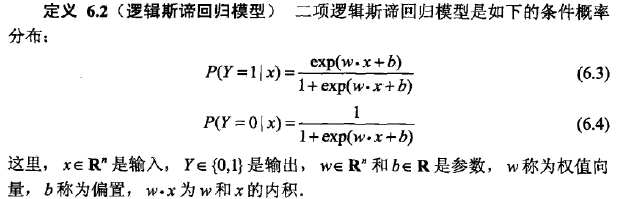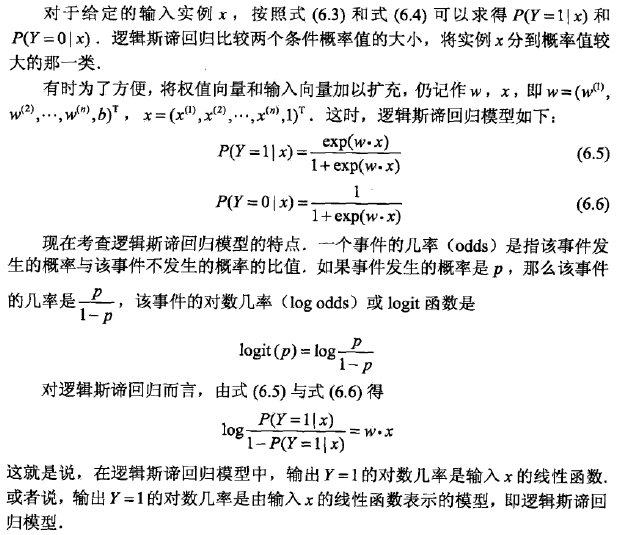线性函数的值越接近正无穷，概率值越接近1，函数的值越接近负无穷，概率值越接近0，这样的模型就是逻辑斯蒂回归模型。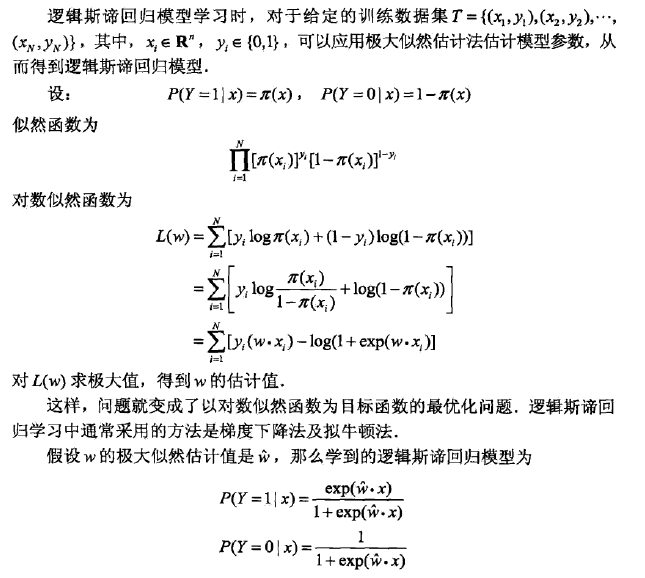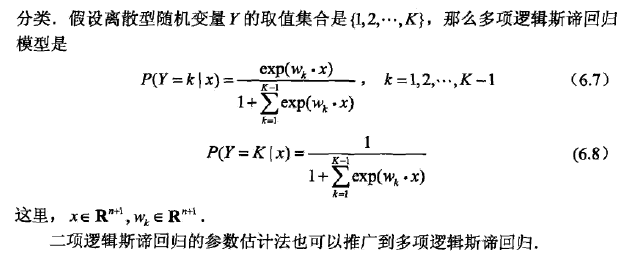最大熵原理
最大熵原理是概率模型学习的一个准则。最大熵原理认为，学习概率模型时，在所有可能的概率模型分布中，熵最大的模型是最好的模型，通常用约束条件确定概率模型的集合。所以，最大熵原理也可以表述为在满足约束条件的模型集合中选取熵最大的模型。（拉格朗日乘数法，写出约束条件，然后分别求导，令其为0）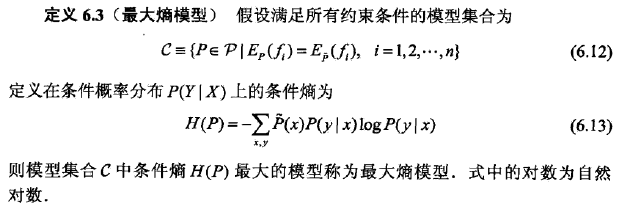最大熵模型更一般的形式：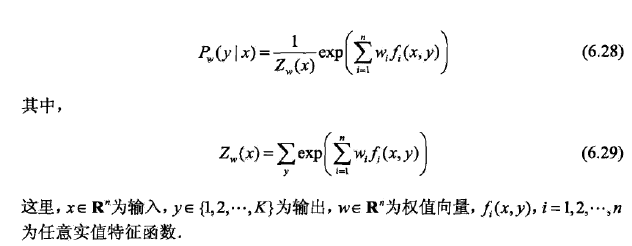改进的迭代尺度法
改进的迭代尺度法（IIS）是一种最大熵模型学习的最优化算法。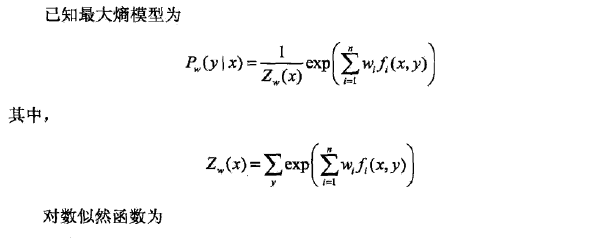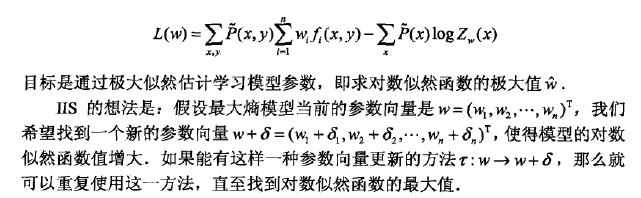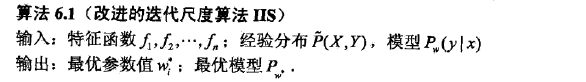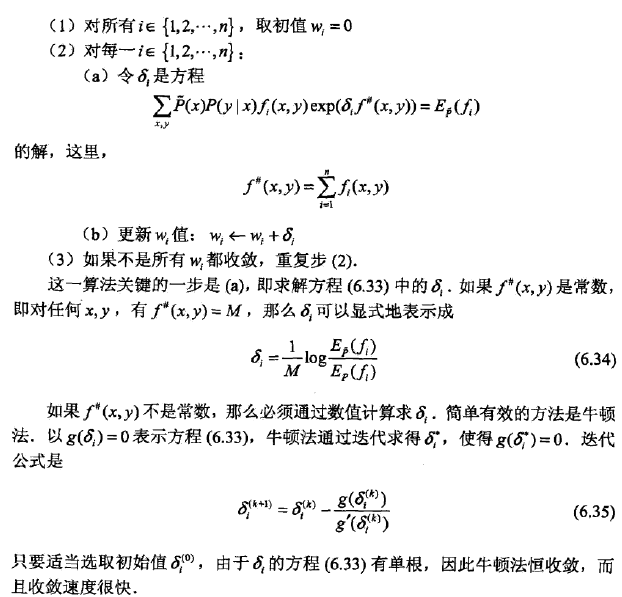拟牛顿法的BFGS算法：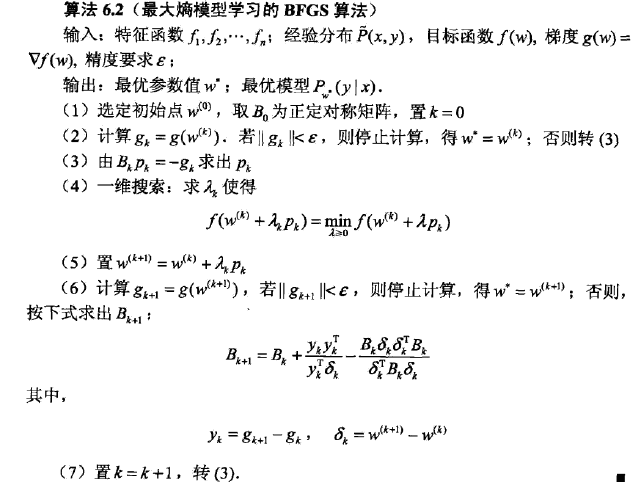展开全文统计学习方法
• .1.8分类问题 1.9标注问题 1.10回归问题 本章概要 继续阅读 习题 参考文献 第2章感知机 2.1感知机模型 2.2感知机学习策略 2.2.1数据集的线性可分性 2.2.2感知机学习策略 2.3感知机学习算法 2.3.1感知机学习算法的...
• 第8-9章介绍了深度学习在计算机视觉领域的目标检测图像分割大应用；第10-14章介绍了计算机视觉领域主要的优化方法思路等，包括迁移学习、网络架构训练、网络优化技巧、超参数调整模型的压缩和加速等。本书...
• 包括计算机视觉基本理论、运动目标检测技术、运动目标跟踪和分类技术、运动的场景分析行为理解技术。其中目标的检测与识别技术在资助的科研项目中有成熟可行的应用实例。本书内容由浅入深、循序渐进，着重于经典...
• CDA数据分析师系列丛书按照数据分析师规范化学习体系而定，对于一名初学者，应该先掌握必要的概率、统计理论基础，包括描述性分析，推断性分析，参数估计，假设检验，方差分析，回归分析等内容，这在本书《从零进阶...
• 寻找新的位置指纹二次特征提取方法采用“软划分”技术方面加以改进。 其中，FC-ID-FKM 法将位置指纹归为区间值数据，在由区间中值和大小张成 的特征空间中，利用模糊k-means 算法对其进行聚类。FC-KFKM 法将位置...室内定位
• 地震传感器参数指标使用事项 有关超声探头阻抗的分析 模拟信号采样与AD转换 设计DSP与FPGA数据采集硬件系统时遇到的一些问题整理 运放参数解释常用运放选型 高频总线上的串阻问题 程序设计 Matlab中的笛卡尔...
• 2.3 参数估计13 2.4 采用混合高斯分布对语音特征建模 15 3 隐马尔可夫模型及其变体17 3.1 介绍17 3.2 马尔可夫链19 3.3 序列与模型 20 3.3.1 隐马尔可夫模型的性质21 3.3.2 隐马尔可夫模型的仿真22 3.3.3 隐...
• 11.3.2 参数估计 11.3.3 对均值矢量的变换 11. 4 MAP算法介绍 11.4.1 MAP算法准则 11.4.2 MAP算法公式推导 11.4.3 MAP算法讨论 11.5 说话人归一化技术 11.5.1 说话人归一化技术原理 11.5.2 声道长度归一化...
• 2 参数估计 　11. 3. 3 对均值矢量的变换 　11. 4 MAP算法介绍 　11. 4. 1 MAP算法准则 　11. 4. 2 MAP算法公式推导 　11. 4. 3 MAP算法讨论 　11. 5 说话人归一化技术 　11. 5. 1 说话人归一化...语音识别
• B题：确定高精度参数问题 C题：维修线性流量阀时的内筒设计问题 D题：学生面试问题 2005年优秀论文 A题：Highway Traveling time Estimate and Optimal Routing B题：空中加油 C题：城市交通管理中的出租车规划 ...
• ## 实用语音识别基础

热门讨论 2013-08-01 19:58:17
2 参数估计 　11. 3. 3 对均值矢量的变换 　11. 4 MAP算法介绍 　11. 4. 1 MAP算法准则 　11. 4. 2 MAP算法公式推导 　11. 4. 3 MAP算法讨论 　11. 5 说话人归一化技术 　11. 5. 1 说话人归一化...语音识别
• §3.4.29 分类区的大小(SORT_AREA_SIZE) 79 §3.4.30 用户卸出文件的路径(USER_DUMP_DEST) 79 §3.5 SQL脚本文件 79 §3.5.1 建立数据字典的脚本 79 §3.5.2 建立附加的数据字典 80 §3.5.3 带“NO”的脚本 81 §...
• 度量学习也称作距离度量学习、相似度学习，通过学习对象之间的距离，度量学习能够用于分析对象时间的关联、比较关系，在实际问题中应用较为广泛，可应用于辅助分类、聚类问题，也广泛用于图像检索、人脸识别等领域。...
• 决定需求量的参数可分为种：运动参数(价格)和转移参数(除价格外的所有影响需求量变化的因素)。假定所有转移参数的值不变，这样一来，就可以直接用需求曲线来表达运动参数(价格P)和需求量之间的二维关系。 需求...
• Language 求已知数据点的拉格朗日插值多项式 Atken 求已知数据点的艾特肯插值多项式 Newton 求已知数据点的均差形式的牛顿插值...DistgshAnalysis 用Fisher类判别法对样本进行分类 MainAnalysis 对样本进行主成分分析